# matlab nts工具的使用

## NARX 简单介绍

• 原理：时间序列预测，用前p天的数据+今天的数据去预测明天的数据。

• 公式：$y_{(n+1)}=f(y_n,y_{n-1},y_{n-p+1},x_n,x_{n-1},x_{n-p+1})$

##　实践

• 准备数据
% 输入数据，这里采用随机数据进行计算，x相当于p，t相当于y
data = rand(100,7)
t=data(:,4)
p=[data(:,1:3),data(:,5:7)]

• 使用nts工具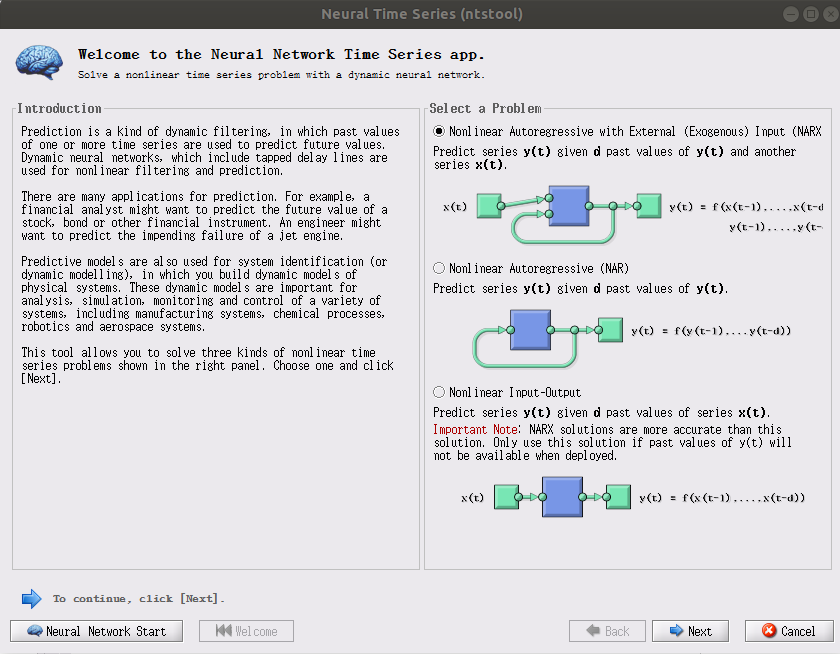• 这里我们选择第一个。

1 NARX $y_{(n+1)}=f(y_n,y_{n-1},y_{n-p+1},x_n,x_{n-1},x_{n-p+1})$ 未来y的结果 和过去的相关x，y都
2 NAR $y_{(n+1)}=f(y_n,y_{n-1},y_{n-p+1})$ 未来y的结果只和y相关
3 NAR $y_{(n+1)}=f(x_n,x_{n-1},x_{n-p+1})$ 未来y的结果只和x相关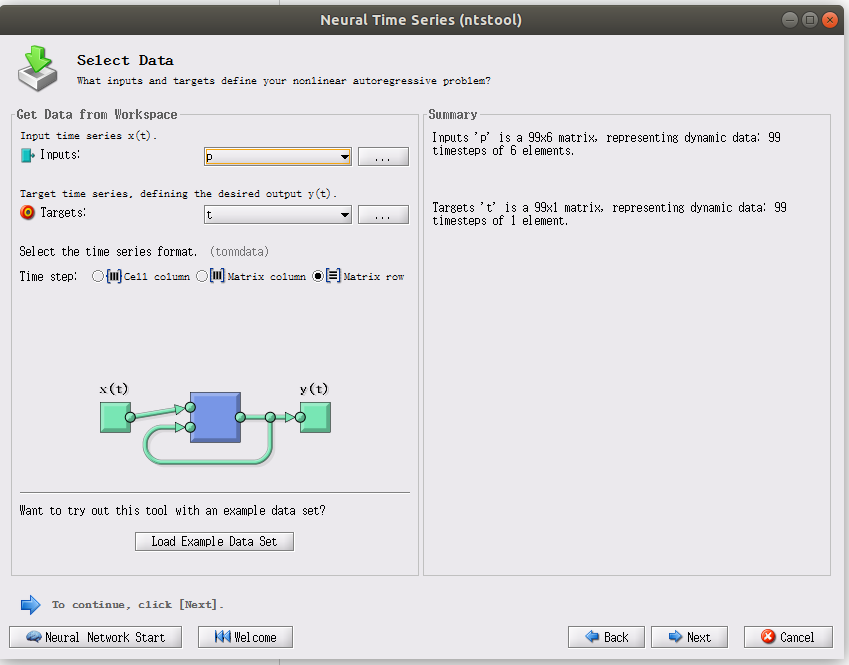• 在这里输入input（x），和target（y）
• 可以看见左下角的图里面matlab会自动帮我们把y重置到输入中，我们之后只需要准备x-y一一对应的数据matlab就会自动帮我们学习并且判断结果。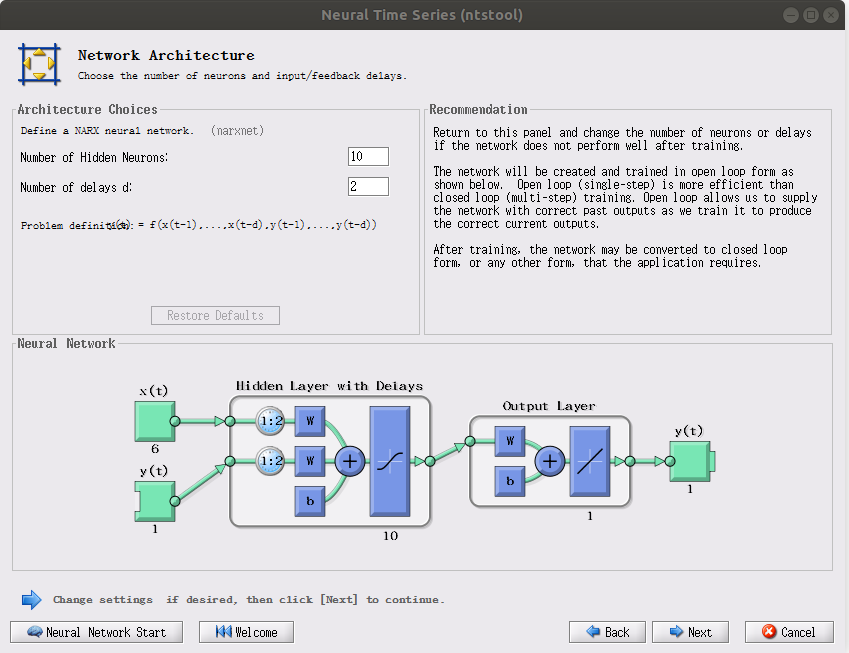number of Hidden Neurons：隐藏层的神经元个数我这里采用默认的
number of delays d ： 用过去第d天的数据来预测未来（也就是之前公式的那个p）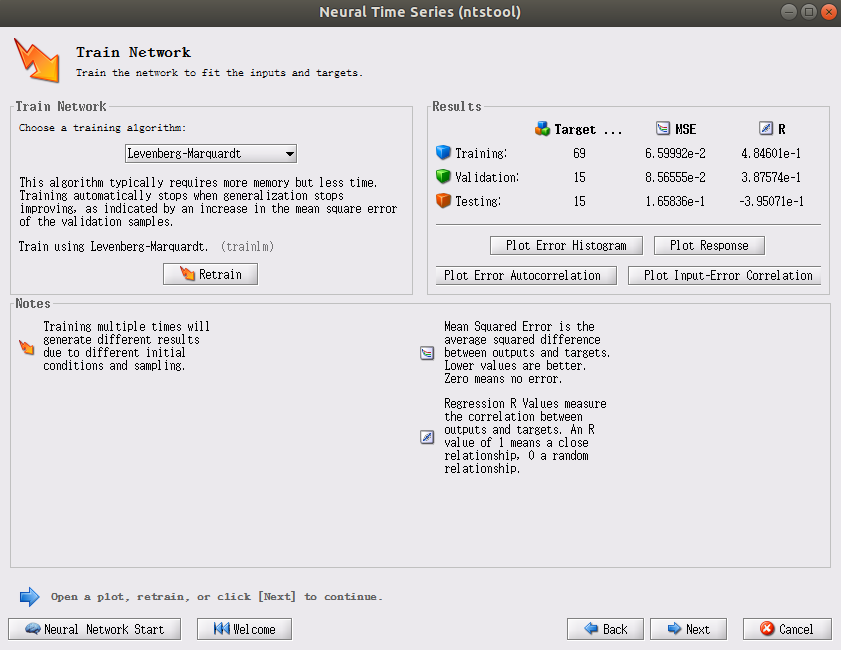• 右上角的MSE越接近0越好，R属于（0,1）越接近1越好（右下角是这样说的）
• 左上角是训练的方式：一般采用levenberg-marquardt（梯度下降），记得点击训练按钮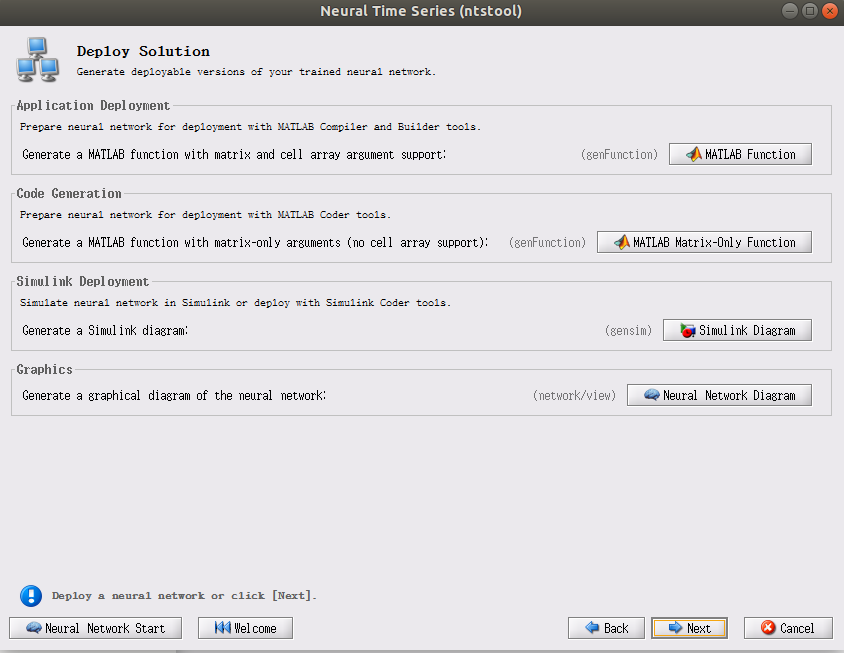• 在这里点击点击第二个MATLAB Matrix-Only Function，会得到一个myNeuralNetworkFunction，如下图所示：（我这里只截取的关键代码，其他的基本不用看）
function [y1,xf1,xf2] = myNeuralNetworkFunction(x1,x2,xi1,xi2)
%MYNEURALNETWORKFUNCTION neural network simulation function.
%
% Generated by Neural Network Toolbox function genFunction, 25-Mar-2020 23:47:50.
%
% [y1,xf1,xf2] = myNeuralNetworkFunction(x1,x2,xi1,xi2) takes these arguments:
%   x1 = 6xTS matrix, input #1
%   x2 = 1xTS matrix, input #2
%   xi1 = 6x2 matrix, initial 2 delay states for input #1.
%   xi2 = 1x2 matrix, initial 2 delay states for input #2.
% and returns:
%   y1 = 1xTS matrix, output #1
%   xf1 = 6x2 matrix, final 2 delay states for input #1.
%   xf2 = 1x2 matrix, final 2 delay states for input #2.
% where TS is the number of timesteps.


• 输入
x1：第n天的x向量
x2：第n天的y向量
xi1：n-p天到n-1天的x向量
yi2：n-p天到n-1天的y向量
• 输出
y1：n+1天预测结果
xf1：和xi1是一个东西，不过这个东西是n-p+1天到第n天的x向量，（用于直接带入n+2天的计算）
xf2：和xi2是一个东西，不过这个东西是n-p+1天到第n天的y向量，（用于直接带入n+2天的计算）©️2019 CSDN 皮肤主题: 大白 设计师: CSDN官方博客